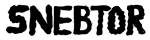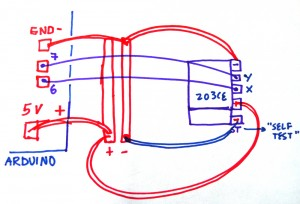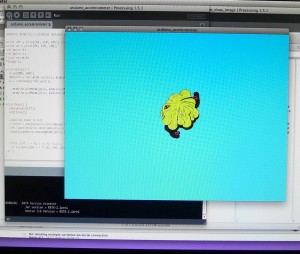### Read values from a 203CE accelerometer directly in Processing1. Upload the “standardFirmata” example from the Arduino IDE in your Arduino board. If you are using Arduino UNO, there is a standard firmata for UNO.

2.Install Arduino library for processing in your libraries folder in the processing sketchbook

3.Test the following code for the Led blink to test that Arduino and processing are communicating correctly (code from the arduino playground page):
``` import processing.serial.*; import cc.arduino.*;```

```Arduino arduino; int ledPin = 13;```

```void setup() { //println(Arduino.list()); arduino = new Arduino(this, Arduino.list(), 57600); arduino.pinMode(ledPin, Arduino.OUTPUT); }```

` ````void draw() { arduino.digitalWrite(ledPin, Arduino.HIGH); delay(1000); arduino.digitalWrite(ledPin, Arduino.LOW); delay(1000);      } ```

DEBUG.Try the analog read program in the arduino and use the serial monitor to see the maximum and minumum values of the accelerometer. You can take my word that they will be from 300 to 700. Supposedly should be (0-1023) in analog read with mid values around (500).

4. If the LED in the Arduino blinks it means that your “hello world” between the Arduino firmata and processing is working.

5.Build the circuit: (Connect to A0 for Y and A1 for X) in diagram it says 6 and 7, but it should be an analog pin.This is the schematic in paper and the photo here:

6. Use the following code in processing:
``` import processing.serial.*; import cc.arduino.*; Arduino arduino; //creates arduino object color back = color(64, 218, 255); //variables for the 2 colors int xpin= 0; int ypin= 1; float value=0; PImage a; void setup() { size(800, 600); arduino = new Arduino(this, Arduino.list(), 57600); //sets up arduino a = loadImage("mar.png"); arduino.pinMode(xpin, Arduino.INPUT);//setup pins to be input (A0 =0?) arduino.pinMode(ypin, Arduino.INPUT); } void draw() { background(back); noStroke(); image(a,arduino.analogRead(xpin)-180, arduino.analogRead(ypin)-300); // in case you want to draw an image //ellipse(arduino.analogRead(xpin)-130, arduino.analogRead(ypin)-130,30, 30); // in case you want an ellipse } ```

–This program tracks down the X and Y values from the accelerometer and displays them altering the position of a graphic in the screen.

–You’ll need to replace the image mar.png in the data folder.

–The position of the object needs to be calibrated to the min and max values the accelerometer is giving you through the serial monitor and for this reason I substract 180 and 330 to the position of the graphic. It still needs improvement!0 Responses to this post